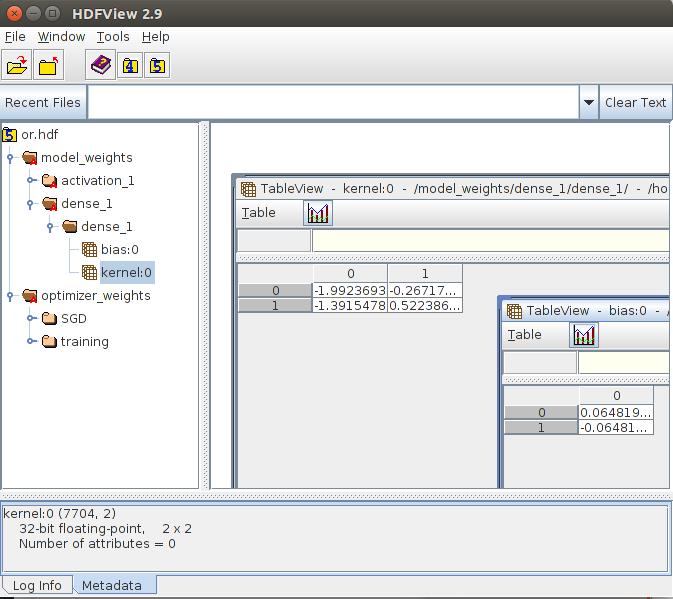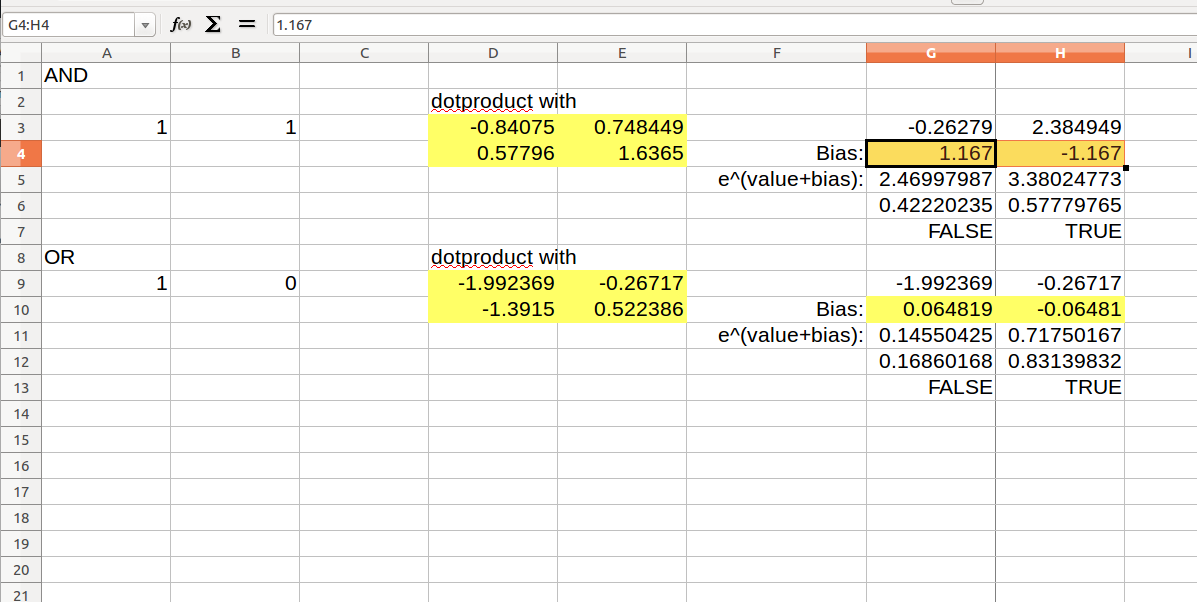# Into Neural Networks! Part 1

Keras is a library that makes machine learning easy to run and train without knowing too much of the math behind it. It has many  tutorials including an excellent howto by Egghead.io, Pyimagesearch and of course the official documentation and books…

…but what if you want to look into the details of how it works? Neural networks are a series of functions that are adjusted over time, and we can “see” what happens in a simple example.

## Making a very basic network

Let’s look at a small neural net, training it on the “OR” function.  Just like the example code in the PyImageSearch dog-vs-cat example, we want output in one of two values – True and False, not Dog/Cat though. Also, there are only two inputs and only one layer is necessary in this simple example:

```from sklearn.preprocessing import LabelEncoder
from sklearn.model_selection import train_test_split
from keras.models import Sequential
from keras.layers import Activation
from keras.optimizers import SGD
from keras.layers import Dense
from keras.utils import np_utils
import numpy as np

data = [[0,0],[0,1],[1,0],[1,1]]
labels = [0,1,1,1]

# encode the labels, converting them from strings to integers
le = LabelEncoder()
labels = le.fit_transform(labels)

data = np.array(data)
labels = np_utils.to_categorical(labels, 2)

# partition the data into training and testing splits?
print("[INFO] constructing training/testing split...")
(trainData, testData, trainLabels, testLabels) = train_test_split(
data, labels, test_size=0.0, random_state=42)
print('train')
print(trainData)
print(trainLabels)
print('test')
print(testData)
print(testLabels)
#In this case test must be train, there are only a few values. copy:
testData = trainData
testLabels = trainLabels

# define the architecture of the network
model = Sequential()

# train the model using SGD
print("[INFO] compiling model...")
sgd = SGD(lr=0.2)
model.compile(loss="binary_crossentropy", optimizer=sgd,
metrics=["accuracy"])
#print(model.fit.__doc__) #print documentation
model.fit(trainData, trainLabels, epochs=50, batch_size=128,
verbose=False)

print(model.predict(np.array([[0,1]])))
print('Should be [0,1]')
print(model.predict(np.array([[1,0]])))
print('Should be [0,1]')
print(model.predict(np.array([[0,0]])))
print('Should be [1,0]')#false
print(model.predict(np.array([[1,1]])))
print('Should be [0,1] true.')
# show the accuracy on the testing set
print("[INFO] evaluating on testing set...")
(loss, accuracy) = model.evaluate(testData, testLabels,
batch_size=128, verbose=1)
print("[INFO] loss={:.4f}, accuracy: {:.4f}%".format(loss,
accuracy * 100))

print("[INFO] dumping architecture and weights to file...")
model.save("or.hdf")
```

Note that the result is saved to a file that you could load later… and you can dig into how it works by reading that hdf file!

(If you didn’t get 100% accuracy when running that, try again and it should make a 100% accurate one after a couple tries, it starts out randomized and may have started with values that won’t work with this small amount of data.)

Note that Ubuntu probably told you to install the “HDFView” to open that file… install it and you will see a collections of numbers in a matrix, something like:Now this matrix is the single layer that was added with

Note that you can do the equivalent in a Spreadsheet:The lower yellow cells correspond to values that you see in the stored model in hdf viewer. Note the dot product, which takes sum of multiplied x’th row and y’th column, and the bias which is added to an exponential – note the softmax function, that is e^x / sum(e^x values) in columns G and H, this normalizes the values to always positive and adding to 1. Although there is little data we can give this network to train, it still says “with 83% certainty” that True and True is True, and chooses the correct false/true value for all 4 possibilities.

## And… there’s more!

You can do the same thing to make an AND function in the same way – note the few changes on the labels, only True and True [1,1] is labeled a True value:

```from sklearn.preprocessing import LabelEncoder
from sklearn.model_selection import train_test_split
from keras.models import Sequential
from keras.layers import Activation
from keras.optimizers import SGD
from keras.layers import Dense
from keras.utils import np_utils
import numpy as np

data = [[0,0],[0,1],[1,0],[1,1]]
labels = [0,0,0,1]

# encode the labels, converting them from strings to integers
le = LabelEncoder()
labels = le.fit_transform(labels)

data = np.array(data)
labels = np_utils.to_categorical(labels, 2)

# partition the data into training and testing splits?
print("[INFO] constructing training/testing split...")
(trainData, testData, trainLabels, testLabels) = train_test_split(
data, labels, test_size=0.0, random_state=42)
print('train')
print(trainData)
print(trainLabels)
print('test')
print(testData)
print(testLabels)
#In this case test must be train, there are only a few values. copy:
testData = trainData
testLabels = trainLabels

# define the architecture of the network
model = Sequential()

# train the model using SGD
print("[INFO] compiling model...")
sgd = SGD(lr=0.2)
model.compile(loss="binary_crossentropy", optimizer=sgd,
metrics=["accuracy"])
#print(model.fit.__doc__) #print documentation
model.fit(trainData, trainLabels, epochs=50, batch_size=128,
verbose=False)

print(model.predict(np.array([[0,1]])))
print('Should be [1,0]')#false
print(model.predict(np.array([[1,0]])))
print('Should be [1,0]')#false
print(model.predict(np.array([[0,0]])))
print('Should be [1,0]')#false
print(model.predict(np.array([[1,1]])))
print('Should be [0,1] true.')
# show the accuracy on the testing set
print("[INFO] evaluating on testing set...")
(loss, accuracy) = model.evaluate(testData, testLabels,
batch_size=128, verbose=1)
print("[INFO] loss={:.4f}, accuracy: {:.4f}%".format(loss,
accuracy * 100))

print("[INFO] dumping architecture and weights to file...")
model.save("and.hdf")

```

So that’s how the neural network calculates – a larger one is going to make a much larger matrix, and a multi-layer is going to have more matrices, but it’s still using the same type of calculation in the spreadsheet!